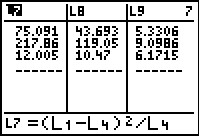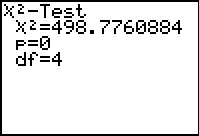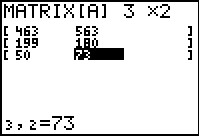••• ##### Device
• TI-83 Plus Family
• TI-84 Plus
• TI-84 Plus Silver Edition
•TI-84 Plus C Silver Edition
•TI-84 Plus CE
• ##### Software

TI Connect™
TI Connect™ CE

# Statistics: Inference for Two-way Tables

by Texas Instruments - Bell Ringer Lesson#### Overview

Students use the chi-square test to analyze whether two categorical variables are independent or dependent calculating expected frequencies, the test statistic and the critical values.

#### Key Steps

•Students will use data from a poll to investigate the independence of the variables in a two-way table. They will use lists to calculate the expected frequencies and the chi-squared test statistic. Then students will compare this value to the critical value, found using a Chi-squared distribution chart.

•Students will use the χ2 –Test command to calculate the P-value. This will confirm students’ decision to reject or fail to reject the null hypothesis of independence.

•A homework problem is included that explores testing the independence of gender and the answer to the poll question. Students can use the list and formulas or the χ2 –Test command as instructed by the teacher.